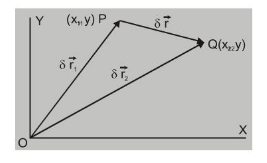Most Affordable JEE | NEET | 8,9,10 Preparation by Kota's Top IITian Doctor Faculties

# What is Displacement ?## Displacement

Displacement is defined as the change in the position of an object. It is a vector quantity and has a direction and magnitude. It is represented as an arrow that points from the starting position to the final position. For example- If an object moves from P position to Q, then the object’s position changes. This change in the position of an object is known as Displacement.

Let the position of point $P$ at time $t_{1}$ be described by position vector $\vec{r}_{1}=x_{1} \hat{i}+y_{1} \hat{j}$ and at time $t_{2}$ position $Q$ is given by position vector $\vec{r}_{2}=x_{2} \hat{i}+y_{2} \hat{j}$from $\Delta \mathrm{OPQ} \Rightarrow \overrightarrow{\mathrm{OP}}+\overrightarrow{\mathrm{PQ}}=\overrightarrow{\mathrm{OQ}}$

or $\quad \overrightarrow{\mathrm{PQ}}=\overrightarrow{\mathrm{OQ}}-\overrightarrow{\mathrm{OP}}$

$\therefore$ Displacement $\overrightarrow{P Q}=\delta \vec{r}=\vec{r}_{2}-\vec{r}_{1}$ in time interval $\delta \mathrm{t}=\left(\mathrm{t}_{2}-\mathrm{t}_{1}\right)$

$\overrightarrow{\delta r}=\left(x_{2} \hat{i}+y_{2} \hat{j}\right)-\left(x_{1} \hat{i}+y_{1} \hat{j}\right)$

$=\left(x_{2}-x_{1}\right) \hat{i}+\left(y_{2}-y_{1}\right) \hat{j}=\delta x \hat{i}+\delta y \hat{j}$

displacement along $X$-axis $\quad \delta x=x_{2}-x_{1}$

displacement along $Y$-axis $\quad \delta y=y_{2}-y_{1}$

Thus displacement in 2 dimensions is equal to vector sum of two one dimensional displacements along mutually perpendicular directions.

Let particle move with uniform velocity $\vec{v}$ at an angle $\theta$ with X-axis.

Then in component form $\vec{v}=v_{x} \hat{i}+v_{y} \hat{j}$

here $v_{x}=v \cos \theta \quad$ and $\quad v_{y}=v \sin \theta$

and $\quad \delta x=v_{x} \delta t \quad, \quad \delta y=v_{y} \delta t$

or $\quad \delta x=(v \cos \theta) \delta t \quad, \quad \delta y=(v \sin \theta) \delta t$

so with $v_{x}$ we get displacement along $X$-axis only and $v_{y}$ gives displacement along $Y$-axis only.

And if particle is moving with uniform acceleration $\vec{a}$, then $\vec{a}=a_{x} \hat{i}+a_{y} \hat{j}$

If direction of $\vec{a}$ makes angle $\phi$ with $X$-axis then $a_{x}=a \cos \phi$ and $a_{y}=a \sin \phi$ are components of $\vec{a}$.

Due to $\mathrm{a}_{\mathrm{x}^{\prime}}$ there is change in $\mathrm{X}$ component of velocity only with no change in $\mathrm{Y}$-component.

Similarly $\mathrm{a}_{y}$ will change only $\mathrm{Y}$ component of velocity at time $\mathrm{t}$

so $\quad v_{x}=u_{x}+a_{x} t \quad$ (here $u_{x}$ and $u_{y}$ are components of initial velocity)

and $\quad v_{y}=u_{y}+a_{y} t$

hence $\quad v_{x} \hat{i}+v_{y} \hat{j}=\left(u_{x}+a_{x} t\right) \hat{i}+\left(u_{y}+a_{y} t\right) \hat{j}$

$=\left(u_{x} \hat{i}+u_{y} \hat{j}\right)+\left(a_{x} \hat{i}+a_{y} \hat{j}\right) t \Rightarrow$ or $\vec{v}=\vec{u}+\vec{a} t$

and similarly component of displacement are

$s_{x}=u_{x} t+\frac{1}{2} a_{x} t^{2}$ and $\mathrm{s}_{\mathrm{y}}=\mathrm{u}_{\mathrm{y}} \mathrm{t}+\frac{1}{2} \mathrm{a}_{\mathrm{y}} \mathrm{t}^{2}$

hence $\quad s_{x} \hat{i}+s_{y} \hat{j}=\left(u_{x} \hat{i}+u_{y} \hat{j}\right)+\frac{1}{2}\left(a_{x} \hat{i}+a_{y} \hat{j}\right) t^{2}$

or $\overrightarrow{\mathrm{s}}=\overrightarrow{\mathrm{u}} \mathrm{t}+\frac{1}{2} \overrightarrow{\mathrm{a}} \mathrm{t}^{2}$